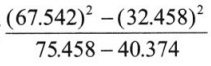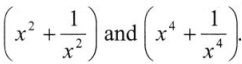# Grade 8 Algebraic Expressions and Identities Worksheets

### Grade 8 Maths Algebraic Expressions and Identities Multiple Choice Questions (MCQs)

1. What are the co-efficients of y in the expression yz2 + 5?
(a) 5
(b) z
(c) z2
(d) None of these

2. The constant term in the expression 1 + x2 + x is
(a) 1
(b) 2
(c) x
(d) x2

3. The expression x + y – xy is:
(a) Monomial
(b) Binomial
(c) Trinomial

4. From the following expressions 3ab, a2, b2, a, 5ab, -2ab, 2a2 the three like terms are:
(a) 3ab, 5ab, -2ab
(b) a2, a, 2a2
(c) 3ab, a2, b2
(d) 2a2, a2, a

5. The value of expression 5n – 2, when n = -2 is:
(a) -12
(b) 8
(c) 1
(d) -8

6. If the length of each side of the equilateral triangle is l, then the perimeter of the equilateral triangle is:
(a) 3l
(b) 3 + 1
(c) 3 – l
(d)$\frac{l}{3}$

7. Which of the following is trinomial:
(a) 2a + 6b – 1
(b) 1
(c) 5a – 7
(d) a + b + c – 3

8. The value of expression 5n2 + 5n – 2 for n = -2 is
(a) 13
(b) 3
(c) 8
(d) 12

9. The expression for the statement: “y multiplied by 10 and then 7 added to product”.
(a) 10 + y + 7
(b) 7y + 10
(c) 10y + 7
(d) 10y

10. The numerical co-efficient of y in the expression 2x + 3y + 7z is:
(a) 2
(b) 3
(c) 7
(d) 3y

11. ‘2’ is common factor of the expressions:
(a) 12a2 b, 15ab2
(b) 5xy, 10x
(c) 10x2, -18x3, 14x4
(d) 33y, -22z

12. The area of a rectangle is ‘xy’ where V is length and ly’ is breadth. If the length of rectangle is increased by 5 units and breadth is decreased by 3 units, the new area of rectangle will be:
(a) (x – y)(x + 3)
(b) xy + 15
(c) (x + 5)(y – 3)
(d) xy + 5 – 3

13. The product of a2, 2a2, 5a10 is
(a) 10 a14
(b) 7 a14
(c) 10 a22
(d) 10 a40

14. Which of the following expression is trinomial:
(a) xyz
(b) xy + z
(c) x + y + z
(d) x + yz

15. Value of expression a(a2 + a + 1) + 5 for ‘ a’ = 0 is:
(a) a + 5
(b) 1
(c) 6
(d) 5

16. Evalute (4x + y)2 by suitable identity:
(a) 4x2 + y2 + 8x
(b) 4x + y + 8xy
(c) 16x2 + y2 + 8xy
(d) 16x2 + y2 + 8

17. (a – b)2 is equal to:
(a) a2 + b2 – 2ab
(b) a2 – b2 + 2ab
(c) a2 – b2
(d) (a – b)(a + b)

18. If ab = 6 and a + b = 5 then the value of (a2 + b2) is:
(a) 11
(b) 12
(c) 13
(d) 16

19. If x – y = 7 and xy = 9, then the value of (x2 + y2) is:
(a) 64
(b) 67
(c) 63
(d) None of these

20. If$\left(x+\frac{1}{x}\right)$ = 4, then the value of$\left(x^{2}+\frac{1}{x^{2}}\right)$ is:
(a) 14
(b) 12
(c) 16
(d) None of these

### Class 8 Maths Algebraic Expressions and Identities Fill In The Blanks

1. The number of terms in the product of (3x2 + 6xy + 5y2) and (3x + 4y – 13) is ……………….. .
2. In the product of (4a3 + 5a2 – 11a) and (-15 + 3a – 7a2), the co-efficient of a4 is …………………… .
3. The value of (3a – 4b + 2) × (5a + 6) for a = 1 and b = -2 is ……………… .
4. The value ofis ………………. .
5. Find the product: (A + B).(A – B).(A2 + B2).(A4 + B4) = ………………….. .

### Class 8 Maths Algebraic Expressions and Identities True(T) Or False(F)

1. If, a +$\frac{1}{a}$ = 7, then a2 +$\frac{1}{a^{2}}$ = 49
2. The product of a binomial and a trinomial will always have 5 terms.
3. (3x2 + 5y2) × (5x5 + 8y3) = 15x7 + 24x2y3 + 25x5y2 + 40y5

### Class 8 Maths Algebraic Expressions and Identities Very Short Answer Type Questions

1. What are the co-efficients of y in the expression 4x – 3 y?
2. What is the statement for the expression 3mn + 5
3. What is the numerical co-efficient of y2 in the expression 2x2y – 15xy2 + 7y
4. What must be subtracted from 2a + b to get 2a – b.
5. What must be added to 3x + y to get 2x + 3y?
6. What should be value of ‘a’ if y2 + y – a equals to 3 for y = 1?

### Class 8 Maths Algebraic Expressions and Identities Short Answer Type Questions

1. What must be added to 7z3 – 11z2 – 129 to get 5z2 + 7z – 92 ?
2. If (3x – 5y) = 10 and xy=5, then find the value of 9x2 + 25y2.
3. If x + y = 9 and xy= 14, find x2 – y2.
4. If 3x + 2y = 12 and xy = 6, find the value of 9x2 + 4y2.

### Class 8 Maths Algebraic Expressions and Identities Long Answer Type Questions

1. Given (x2 + y2) = 74 and xy = 35, find the value of x + y and x – y.
2. If x +$\frac{1}{x}$ = 4, find the value of3. Using suitable identities evaluate the following:
(i) (93)2
(ii) (12.5)2 – (7.5)2
(iii) 93 × 109